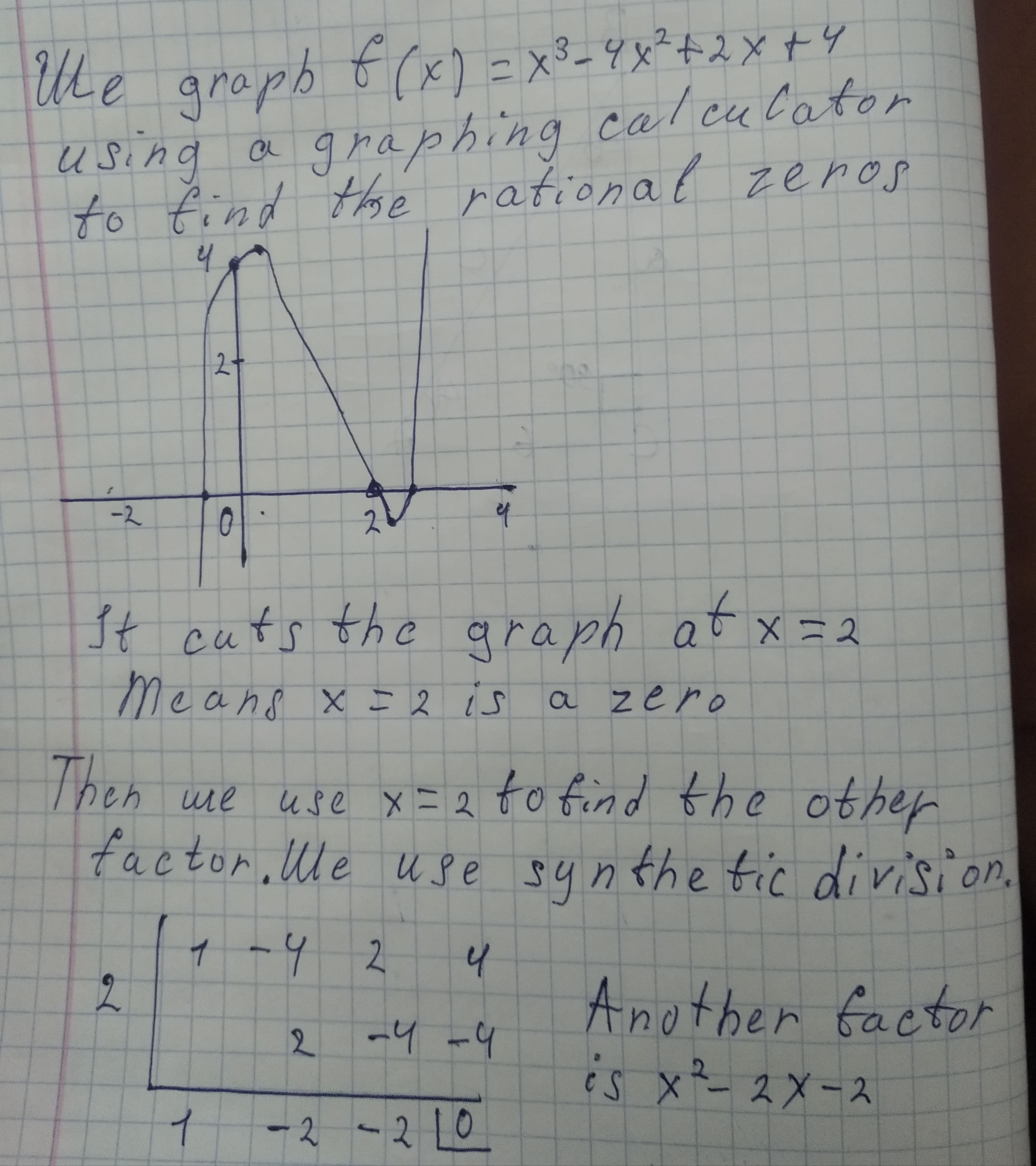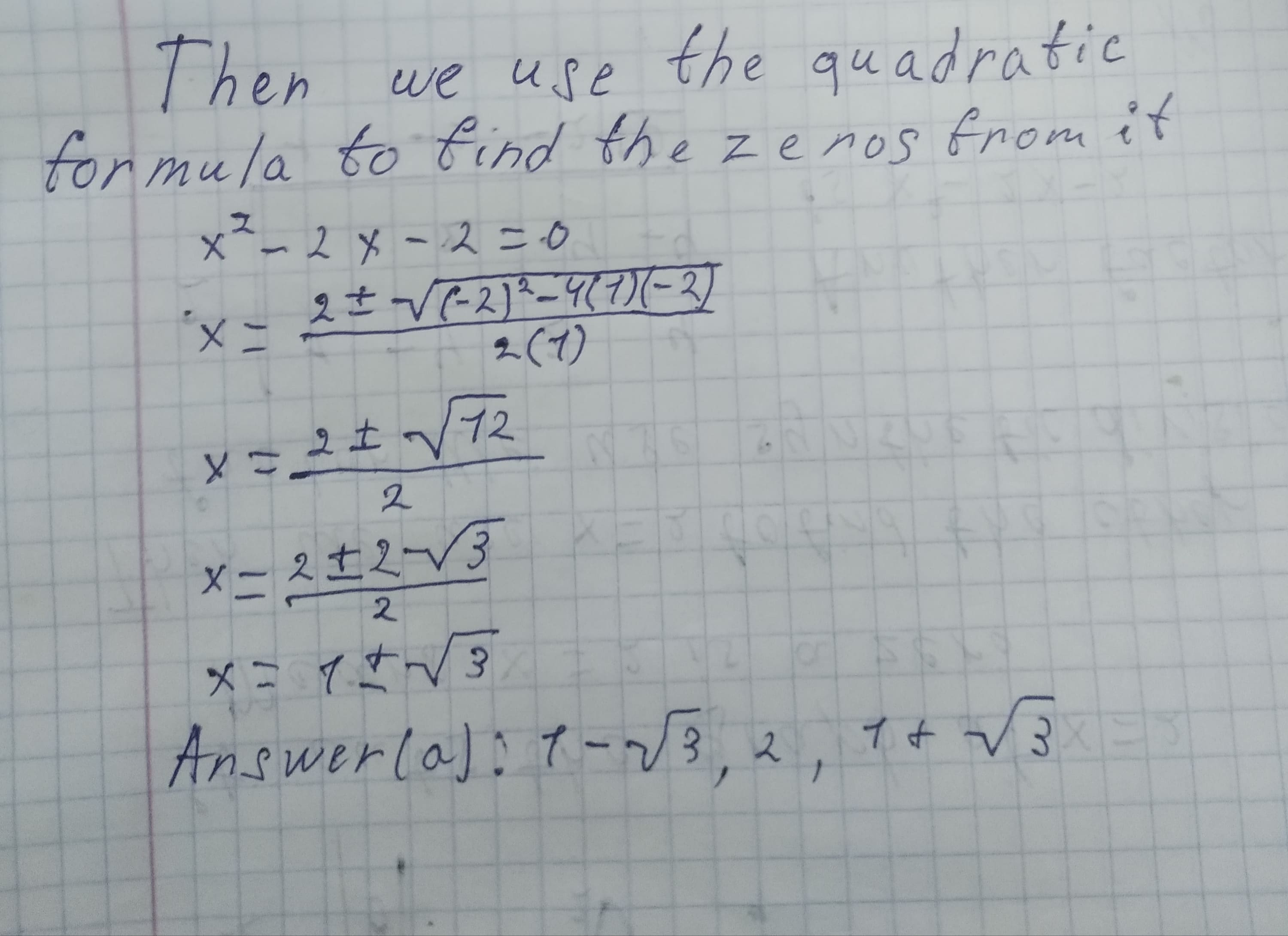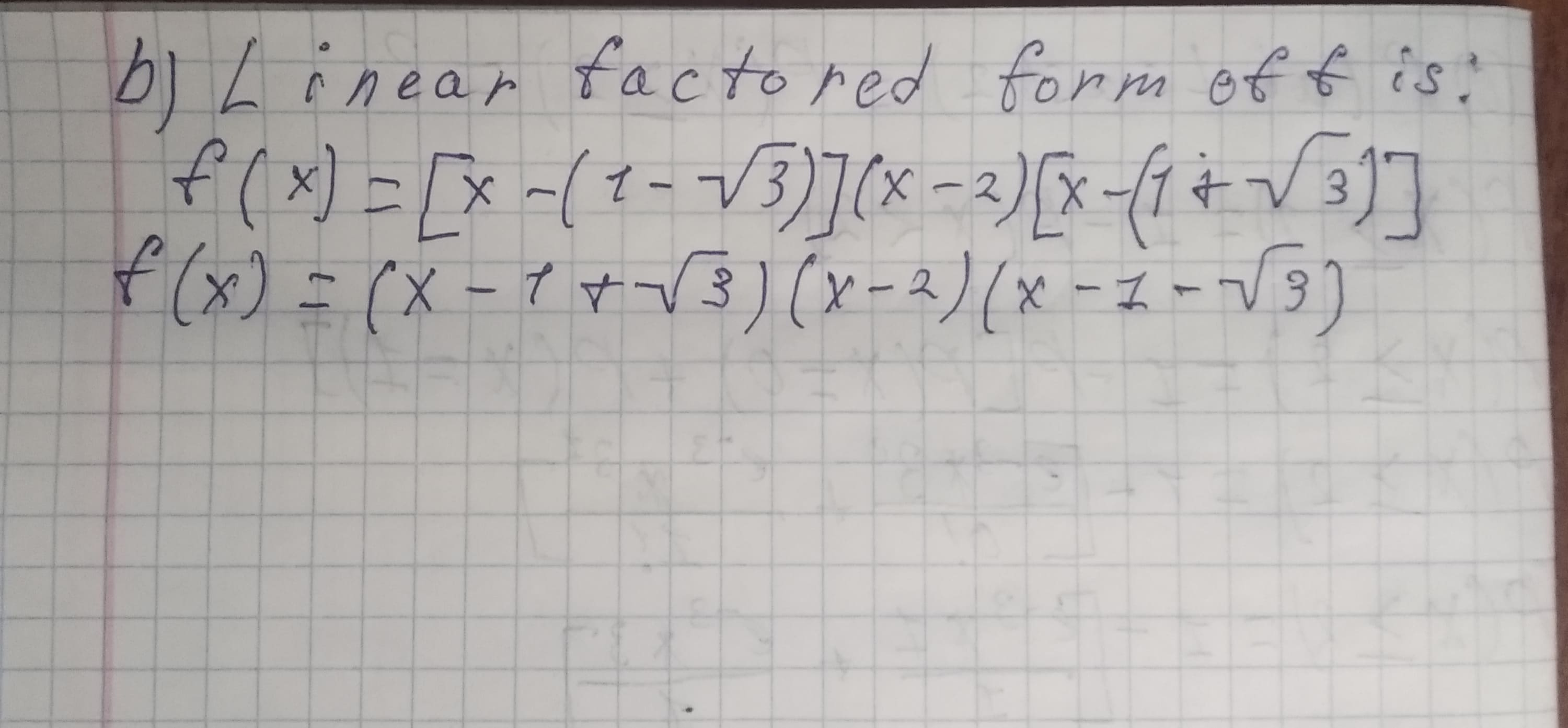(a) find the rational zeros and then the other zeros of the polynomial function (x)=x3-4x2+2x+4, that is, solve f(x)=0. (type an exact answer, using rDottie Parra 2020-12-24 Answered

(a) find the rational zeros and then the other zeros of the polynomial function $$(x)=x3-4x^2+2x+4$$, that is, solve
$$f(x)=0.$$ (type an exact answer, using radicals as needed. Simplify your answer. Use a comma to separate answers as needed.)
(b) Factor f(x) into linear factors. (type an exact answer, using radicals as needed. Simplify your answer. Type an expression using x as the variable.)

• Questions are typically answered in as fast as 30 minutes

Solve your problem for the price of one coffee

• Math expert for every subject
• Pay only if we can solve itSoosteethicU

a) We graph $$f(x) = x^{3} - 4x^{2} + 2x + 4$$ using a graphing calculator to find the rational zeros.It cuts the graph at $$x = 2$$
Means $$x = 2$$ is a zero.
Then we use $$x=2$$ to find the other factor. We use synthetic division.
Another factor is $$x^{2}-2x-2.$$
Then we use the quadratic formula to find the zeros from it
$$x^{2} - 2x - 2 = 0$$
$$x=\frac{2 \pm\sqrt{(-2)^{2}-4(1)(-2)}}{2(1)}$$
$$x=\frac{2 \pm \sqrt{12}}{2}$$
$$x=\frac{2 \pm 2 \sqrt{3}}{2}$$
$$x=1 \pm \sqrt{3}$$
Answer $$(a): 1 -\sqrt{3},2,1+\sqrt{3}$$b) Linear factored form of f is:Answer: $$(x-1+\sqrt{3})(x-2)(x-1-\sqrt{3})$$